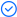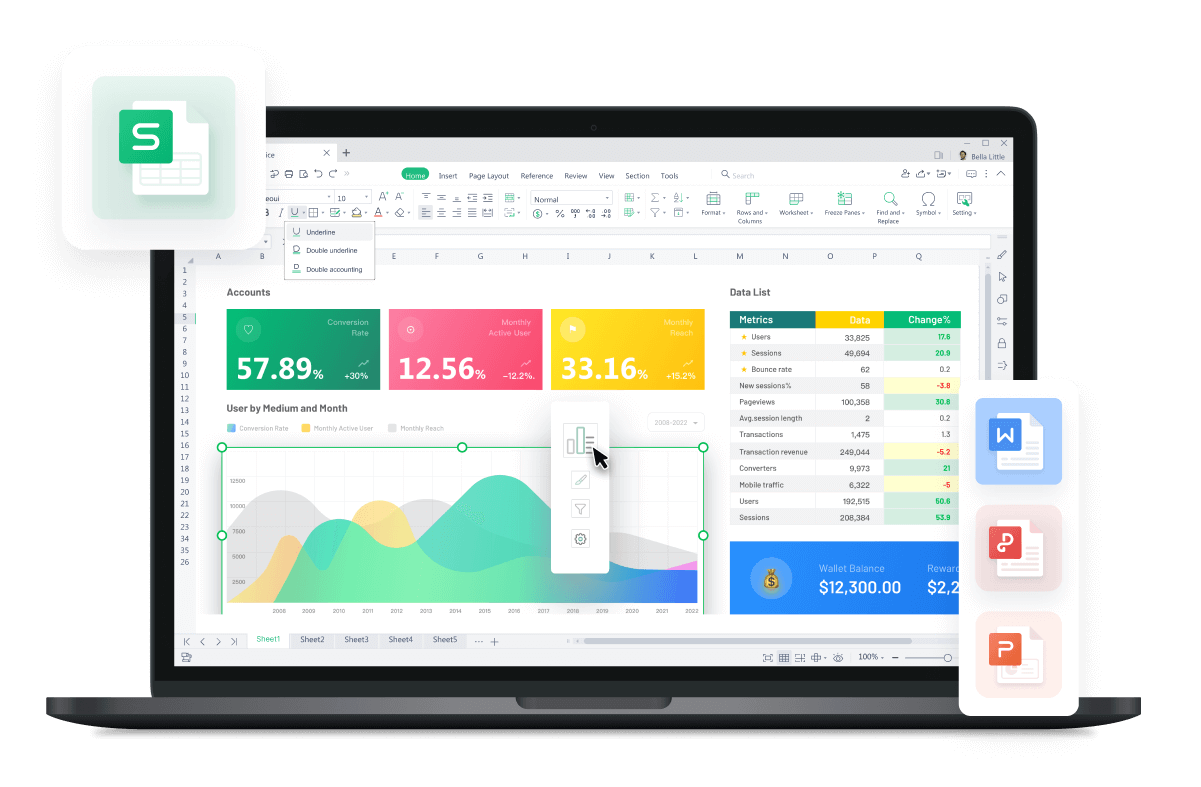WPS Office

Free All-in-One Office Suite with PDF EditorRead, edit, and convert PDFs with the powerful PDF toolkit.Microsoft-like interface, easy to use.

Windows • MacOS • Linux • iOS • Android# DATEDIF function in EXCEL

January 13, 2022
6.4K Views

· Description:

Except for DAYS360 function(hyperlink), there is another more powerful function to calculate dates that are not in the same year. The DATEDIF function is mainly used to countthe number of days, months or years between two dates.

· Syntax:

DATEDIF(start_date, end_date, comparison unit)

· Arguments:

Start_date: A serial date number that represents the start date.

End_date: A serial date number that represents the end date.

Comparison unit: The type of result you want to return.

 Interval Explanation Y The number of years. M The number of months. D The number of days. MD The difference between the days (ignore months and years). YM The difference between the months (ignore days and years). YD The difference between the days (ignore years and dates).

· Example1:

Let's start with some simple cases by using the TODAY function. Suppose we want to calculate employees' length of service of a company, how can we make use of the DATEDIF function?

1. Select cell D3 where we want to calculate how long have mike work. Then head to the Formulas tab > Insert Function > enter DATEDIF in the edit box, or just type =DATEDIF in the cell.

2. Here we need to enter the DATEDIF function.

Start_date: A serial date number that represents the start date.C2 represents the entry date, so we put that at start_date,

End_date: A serial date number that represents the end date.TODAY()return the current time based on our system date.(2021/12/13 when writing this tutorial), so we put it here.

Comparison unit: The type of result you want to return.Y regulate the unit of our returned value, in this case, we put that because the length of service is measured by year.

So, enter C2 at start_date, TODAY() at end_date, Y at Comparison unit. Or type the following function directly in cell D2.

=DATEDIF(C2,TODAY(),Y)

Finally, we get a 3 as our result, which means James has work for 3 years in this company.

3. Hove over cell D2 and drag it all the way down to complete D3 to D16. Up to this point, we have turned the table into what we want.

· Example2:

Suppose we don't want to prepare gifts for every employee in our company. We can use the nested IF & DATEDIF function.

1. Select cell C3, then insert the following formula:

=IF(DATEDIF(C2,TODAY(),YD)

Here is why we write this formula:

1) For the inside DATEDIF function,

1.1 Start_date: A serial date number that represents the start date.C2 represents the start date, so we put it here.

1.2 End_date: A serial date number that represents the end date. TODAY() return the current time based on our system date.(2021/12/13 when writing this tutorial), so we put it here.

1.3 Comparison unit: The type of result you want to return.YD help us to get the difference between the days (ignore years and dates).

2) IF function can check whether a condition is met, and returns one value if TRUE, and another value if FALSE. In this case, it helps us to judge whether the difference is within 7 days, that is what we write in DATEDIF. If so, it will return YES, otherwise, it will returnan empty value.

Thus, DATEDIF can return the gap between today and the birthday of  James. Cell D2 return empty value because He won't have a birthday within seven days.

3. Hove over cell D2 and drag it all the way down to complete D3 to D16. Up to this point, we can see from the table that it's time for the HR department to prepare gifts for Alex and Andrew.